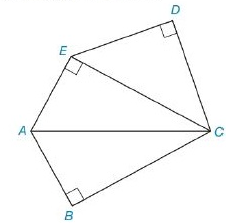Chapter 8.2, Problem 29E### Elementary Geometry for College St...

6th Edition
Daniel C. Alexander + 1 other
ISBN: 9781285195698

#### Solutions

Chapter
Section### Elementary Geometry for College St...

6th Edition
Daniel C. Alexander + 1 other
ISBN: 9781285195698
Textbook Problem
1 views

# In Exercises 27 to 30, find the area of the figure shown. Given: Pentagon ABCDEwith D C - ≅ D E - A E = A B = 5 B C = 12 Find: A A B C D ETo determine

To Find:

Area of the pentagon provided.

Explanation

Formula Used:

Split the pentagon into three triangles and find the corresponding areas and then sum it up.

Pythagorean theorem for the right angle triangle ABC for the hypotenuse AC,

AC2=AB2+BC2.

Area of triangle =12bh.

Calculation:

Let the vertices of the provided pentagon be A,B,C,D and E.

Let’s divide the pentagon into three triangles. That is, CDE, ACE and ABC.

It is given that mCDE=mCBA=mCEA=90°,  DC-DE-, AE=AB=5 and BC=12.

Consider the triangles ACE and ABC,

Here AE=AB=5 and both the triangles have common base. They are similar triangles. Therefore, the side EC must be equal to BC. That is, EC=BC. As the triangles have same side lengths, their areas will also be same.

Area of ΔABC=12bh=12×5×12=30

### Still sussing out bartleby?

Check out a sample textbook solution.

See a sample solution

#### The Solution to Your Study Problems

Bartleby provides explanations to thousands of textbook problems written by our experts, many with advanced degrees!

Get Started